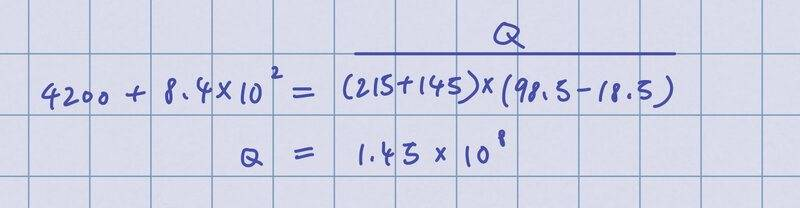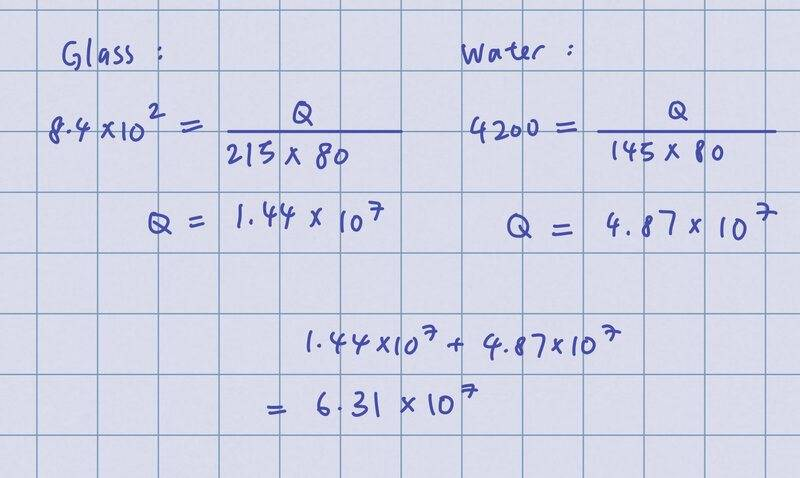# Thermal physics question: Specific heat capacity

• Noawun

#### Noawun

Homework Statement
A glass beaker of mass 215g contains 145g of water at 18.5 degrees Celsius. If the specific heat capacity of glass is 8.4 x 10^2 J kg^-1 K^-1, how much heat energy would need to be supplied to raise the temperature of the glass and water to 98.5 degrees Celsius?
Relevant Equations
Q=mc\Delta T
At first, I tried to calculate the heat energy required by doing this:I realized I should calculate heat energy separately instead of grouping glass and water together so I did this:But the answer is supposed to be 6.29 x 10^4.

I don't know how to solve this. Can anyone help please? Thank you

Homework Statement:: A glass beaker of mass 215g contains 145g of water at 18.5 degrees Celsius. If the specific heat capacity of glass is 8.4 x 10^2 J kg^-1 K^-1, how much heat energy would need to be supplied to raise the temperature of the glass and water to 98.5 degrees Celsius?
Relevant Equations:: Q=mc\Delta T

At first, I tried to calculate the heat energy required by doing this:
View attachment 295998
I realized I should calculate heat energy separately instead of grouping glass and water together so I did this:

View attachment 295999

But the answer is supposed to be 6.29 x 10^4.

I don't know how to solve this. Can anyone help please? Thank you
I get 63168, rounding to 63200. I suspect someone made the error of rounding intermediate values.

It also looks like he's mixed units (g vers kg) which gives the 10^3 difference in his answer? (I didn't check his work in detail, though)

It also looks like he's mixed units (g vers kg) which gives the 10^3 difference in his answer? (I didn't check his work in detail, though)
Well spotted! Looking only at the last line, I misread the handwritten exponent 7, with its continental centre line and the graph paper line at the left, as a four.

•berkeman